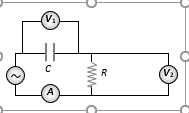Phasors and Phasor Diagrams
Question

# The diagram shows a capacitor C and a resistor R connected in series to an ac source. V1  and V2  are voltmeters and A is an ammeterConsider now the following statementsI. Readings in A and ${V}_{2}$ are always in phaseII. Reading in ${V}_{1}$ is ahead in phase with reading in ${V}_{2}$III. Readings in A and ${V}_{1}$ are always in phase Which of these statements are/is correct

Moderate
Solution

## In RC series circuit voltage across the capacitor lags the voltage across the resistance by   $\frac{\pi }{2}$

Get Instant Solutions